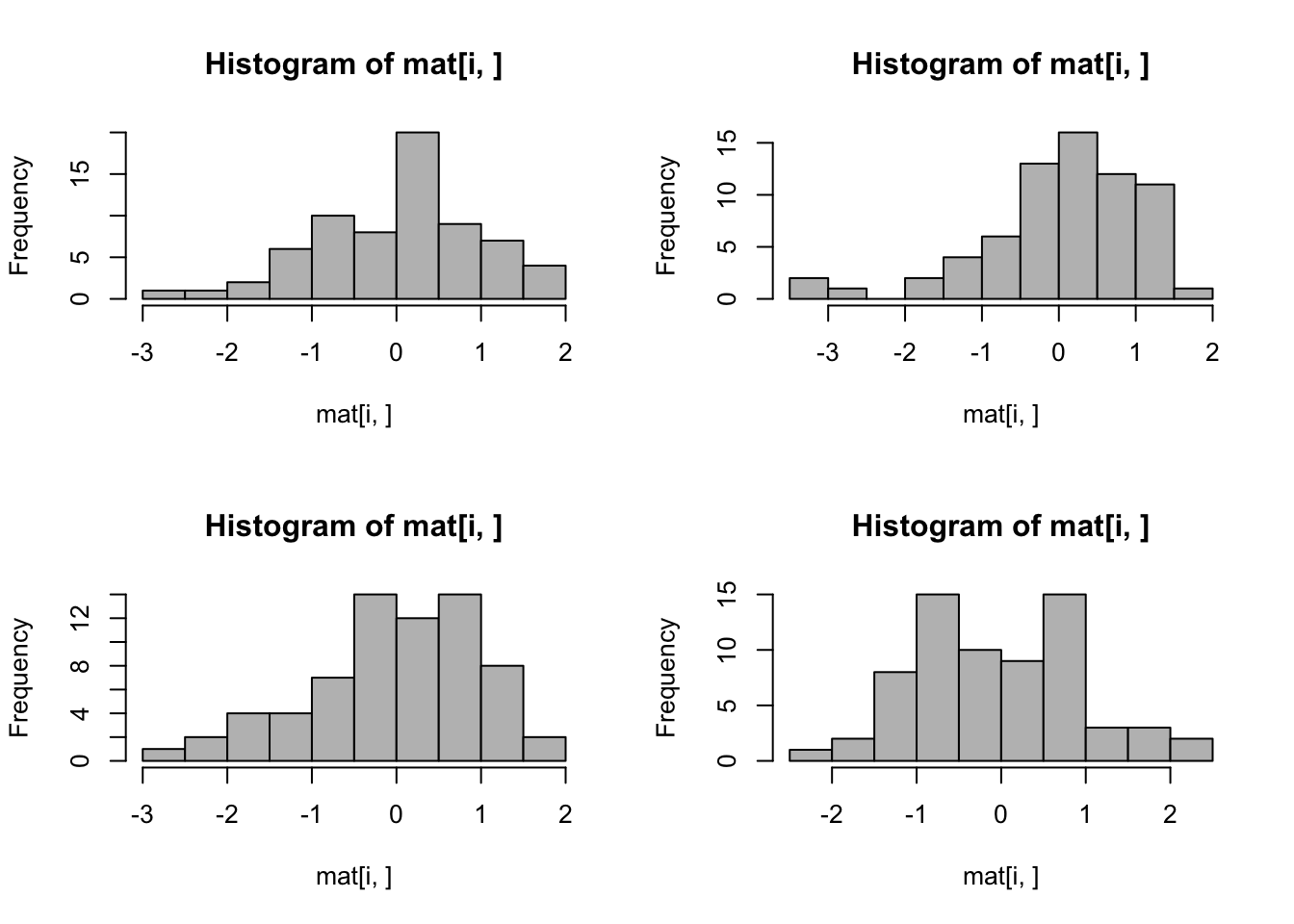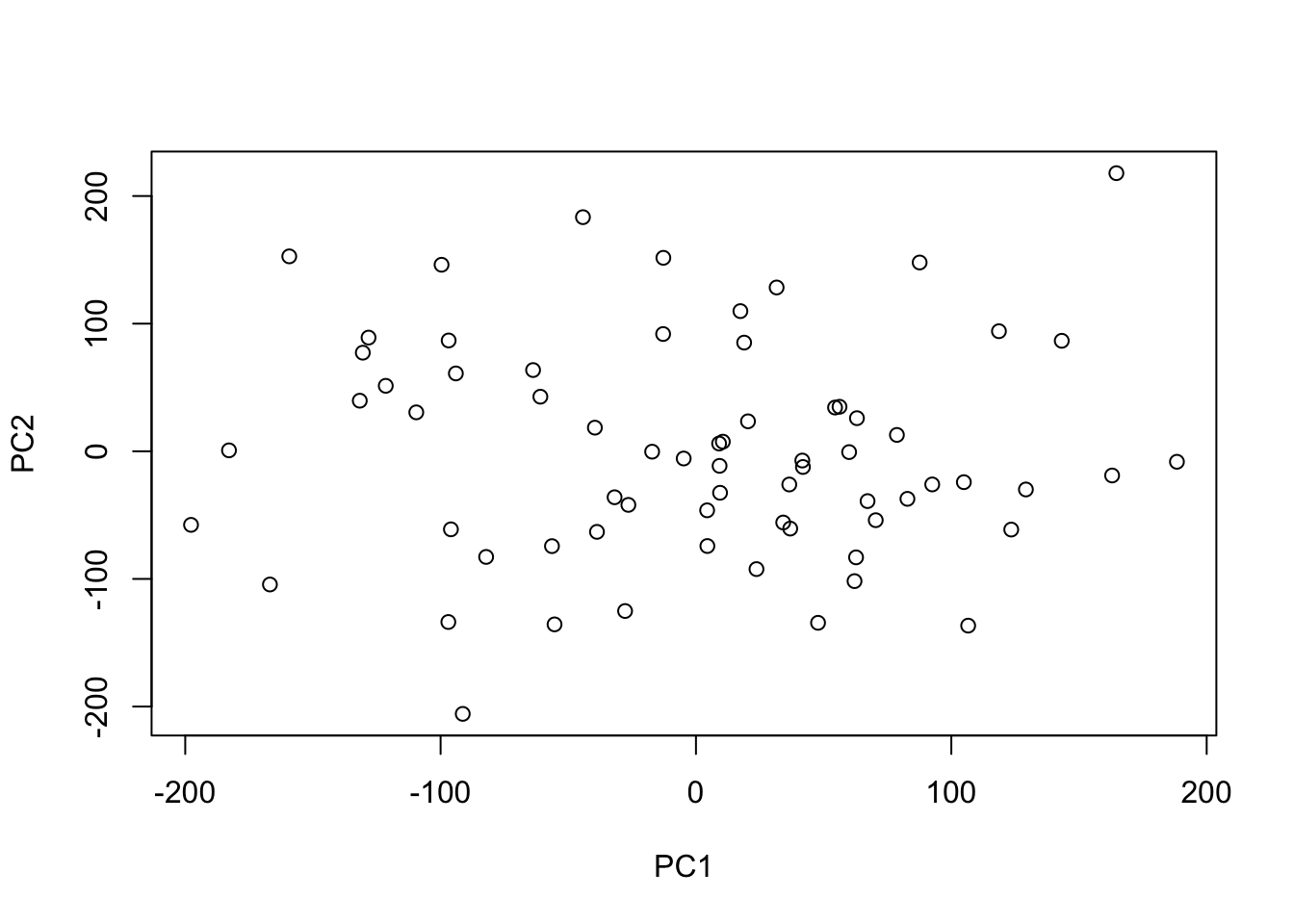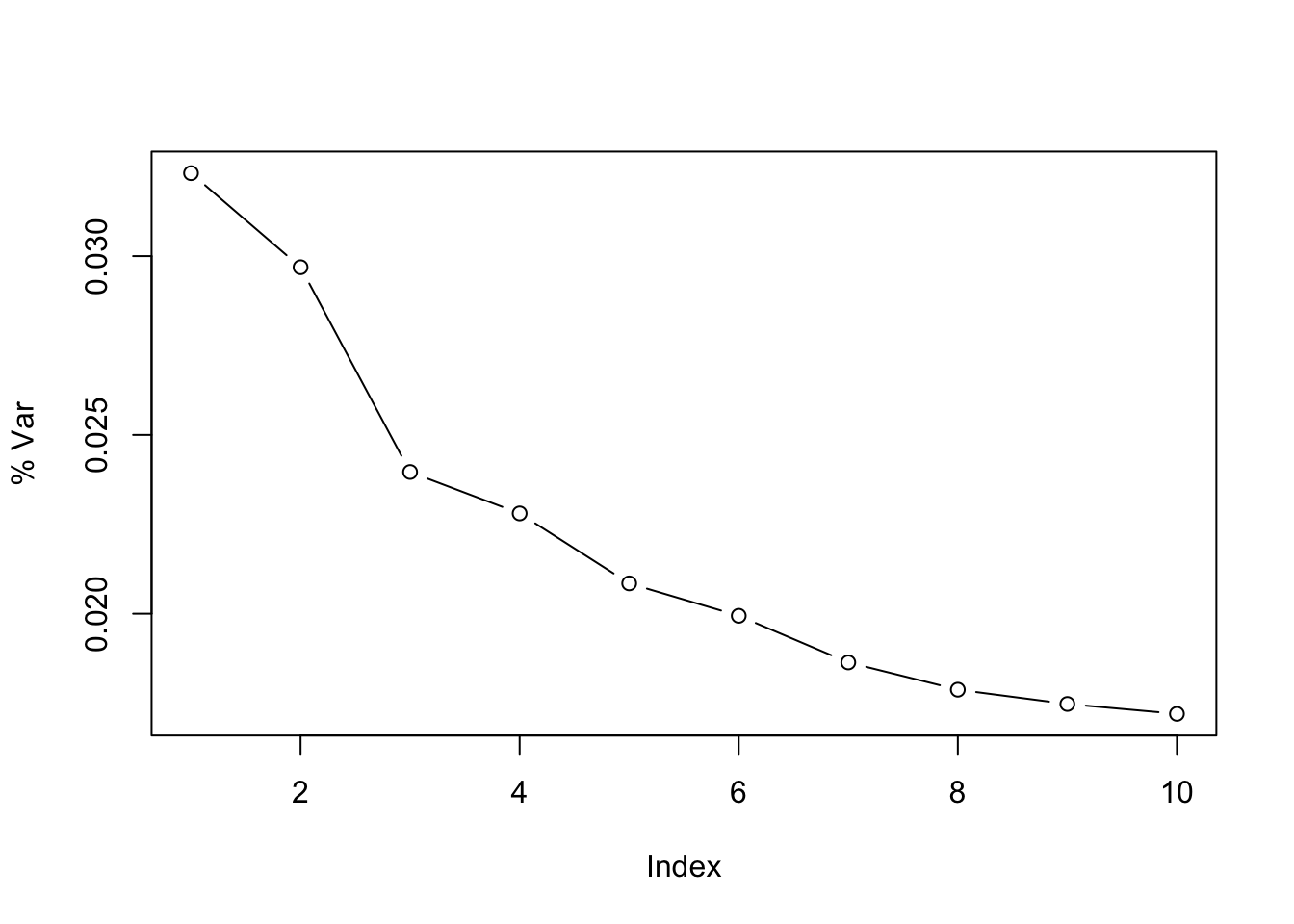Here I will introduce a popular statistical framework for working with the results of many parallel statistical tests, a setting that occurs frequently in genomic analyses. I won’t go into depth here, but will discuss more in lecture. For background you can also read the lecture notes from the HarvardX Biological Data Science series (also the Wikipedia article on multiple comparisons is a good introduction).

While up to this point, we have minimized the use of simulation data to show concepts in Computational Biology, multiple testing is often introduced using simulation data, and there is a practical reason why. We can use real biological datasets to generate test statistics from the null hypothesis, by taking a large group of samples and randomly dividing into two groups with no known biological difference. Unless we happen to choose a partition which corresponds to biological or technical factors, this is pretty good for observing null test statistics. And we can limit this probability by choosing a large dataset, or by balancing our random partition with respect to technical factors, or by removing technical factors using factor analysis methods such as SVA, RUV, or PEER.

However, it’s not easy to generate many test statistics from real data which combine known null and alternative hypotheses, because we often do not know which hypotheses are truly null, e.g. \(\Delta = 0\), and which are alternative, \(\Delta \ne 0\). So we often resort to simulation, or a combination of real null data with simulation used to create alternative hypotheses, to demonstrate the concepts of multiple test correction. Here we will use the latter, starting with a real dataset where we can create a null distribution of test statistics.

# About the dataset

We start by downloading a large, normalized matrix of values for chromatin accessibilty across 68 human donors. Chromatin accessibility refers to a quantitative measurement of whether the DNA at a certain location is packaged tightly or in a more open pattern, which indicates the degree to which proteins can access and bind to DNA – in particular regulatory proteins which might affect transcription of genes. A diagram of inaccessible and accessible chromatin can be found on this Wikipedia page.

The dataset is hosted at this website, and we download a text file of normalized data contained in this subfolder, which is about 130 Mb, compressed. The publication associated with this dataset is listed at the above link, and the goal was to find genetic variants which are associated with differences in chromatin accessibility. We won’t discuss here exactly how the raw data was processed to produce the normalized matrix above, but for our purposes, all we need to know is that large values correspond to more accessible chromatin, each column corresponds to a different human donor, and each row corresponds to a distinct location in the genome. The rows are actually peaks of accessibility that were detected in at least some subset of the samples.

We have to use this `skip=1` argument below because the first row contains only column names, but is missing a column name for the first column (the index number of the peak from a larger set of peaks).

``````url <- "http://chromovar3d.stanford.edu/QTLs/correctedSignal/hMat.norm.ALL.dhs.peer_lS_5.txt.gz"
file <- "hMat.norm.ALL.dhs.peer_lS_5.txt.gz"
dnase <- read_delim(file, delim=" ", skip=1, col_names=FALSE)``````
``````## Parsed with column specification:
## cols(
##   .default = col_double(),
##   X1 = col_integer()
## )``````
``## See spec(...) for full column specifications.``
``dnase``
``````## # A tibble: 250,000 x 69
##       X1          X2         X3         X4         X5          X6
##    <int>       <dbl>      <dbl>      <dbl>      <dbl>       <dbl>
##  1     2 -2.07939782 -1.0919890  0.4358841  1.0771965  0.18202807
##  2     3  1.04012044 -0.6540832  0.5439511  0.5433546  0.33554692
##  3     5  0.61603730  1.6216130 -1.9550178  0.8038654 -1.17296933
##  4     6 -0.08366167  0.1037396 -0.7161806 -0.4290121 -1.05492685
##  5     7  0.71635444 -1.5309856  0.1680760 -1.3674195  0.18572840
##  6     8  0.21563757 -0.1195649 -0.4001214  0.1126866  1.67232404
##  7    16 -1.62173082 -1.9355604  1.5993482 -0.1015715  0.41055978
##  8    17  0.37951245 -1.4808371 -0.0526740 -0.9285295  0.25286624
##  9    18  1.70117468  0.3848198 -0.3243192  1.0260985  0.44060164
## 10    19 -1.94900586 -0.9384677 -1.2035325 -0.2577110  0.08515044
## # ... with 249,990 more rows, and 63 more variables: X7 <dbl>, X8 <dbl>,
## #   X9 <dbl>, X10 <dbl>, X11 <dbl>, X12 <dbl>, X13 <dbl>, X14 <dbl>,
## #   X15 <dbl>, X16 <dbl>, X17 <dbl>, X18 <dbl>, X19 <dbl>, X20 <dbl>,
## #   X21 <dbl>, X22 <dbl>, X23 <dbl>, X24 <dbl>, X25 <dbl>, X26 <dbl>,
## #   X27 <dbl>, X28 <dbl>, X29 <dbl>, X30 <dbl>, X31 <dbl>, X32 <dbl>,
## #   X33 <dbl>, X34 <dbl>, X35 <dbl>, X36 <dbl>, X37 <dbl>, X38 <dbl>,
## #   X39 <dbl>, X40 <dbl>, X41 <dbl>, X42 <dbl>, X43 <dbl>, X44 <dbl>,
## #   X45 <dbl>, X46 <dbl>, X47 <dbl>, X48 <dbl>, X49 <dbl>, X50 <dbl>,
## #   X51 <dbl>, X52 <dbl>, X53 <dbl>, X54 <dbl>, X55 <dbl>, X56 <dbl>,
## #   X57 <dbl>, X58 <dbl>, X59 <dbl>, X60 <dbl>, X61 <dbl>, X62 <dbl>,
## #   X63 <dbl>, X64 <dbl>, X65 <dbl>, X66 <dbl>, X67 <dbl>, X68 <dbl>,
## #   X69 <dbl>``````
``dim(dnase)``
``##  250000     69``
``````col.names <- names(read.delim(file, nrow=1, sep=" "))
col.names``````
``````##   "NA19160" "NA18861" "NA18862" "NA18909" "NA18907" "NA18517" "NA19119"
##   "NA19116" "NA19114" "NA19171" "NA18870" "NA18486" "NA19206" "NA19207"
##  "NA19204" "NA19203" "NA19200" "NA19201" "NA19209" "NA19141" "NA19140"
##  "NA19143" "NA19144" "NA19147" "NA18498" "NA18499" "NA19099" "NA19098"
##  "NA19093" "NA19092" "NA18520" "NA18522" "NA19152" "NA19153" "NA19257"
##  "NA19159" "NA19239" "NA19238" "NA19127" "NA19225" "NA19222" "NA19223"
##  "NA19130" "NA19131" "NA18516" "NA18511" "NA18510" "NA19137" "NA19138"
##  "NA18519" "NA18507" "NA19190" "NA19210" "NA18858" "NA18859" "NA18913"
##  "NA18912" "NA18916" "NA18852" "NA18853" "NA18855" "NA18856" "NA18502"
##  "NA18501" "NA18504" "NA18505" "NA18508" "NA19101"``````

We have 250k peaks and 68 donors. We add the column names that we skipped before:

``````mat <- as.matrix(dnase[,-1])
dim(mat)``````
``##  250000     68``
``colnames(mat) <- col.names``

I take a quick look at the histogram of values for the first four peaks:

``````par(mfrow=c(2,2))
for (i in 1:4) hist(mat[i,], col="grey")``````Also, it’s always a good idea to make a PCA plot of the samples, to look for any large-scale structure in the data:

``````pc <- prcomp(t(mat))
plot(pc\$x[,1:2])``````If you square the standard deviation values and sum them, you get the total variance in the data. Here I show that the top PC is not a large percent of the variance in the data, which means that there is not a low dimensional structure to the data, which one might see with strong batch effects or distinct biological conditions.

``plot(pc\$sdev[1:10]^2 / sum(pc\$sdev^2), type="b", ylab="% Var")``# Multiple testing

To investigate multiple testing, we first generate a mock comparison: I choose two random groups of the data, indicated by a factor variable called `fake` and perform row-wise t-tests across all the 250k peaks in the data. I then look at the distribution of p-values across 20 bins defined on [0,1].

``````set.seed(1)
fake <- factor(sample(rep(1:2,each=ncol(mat)/2)))
library(genefilter)
ts <- rowttests(mat, fake)
nbins <- 20
brks <- 0:nbins/nbins
hist(ts\$p.value, col="grey", breaks=brks)``````